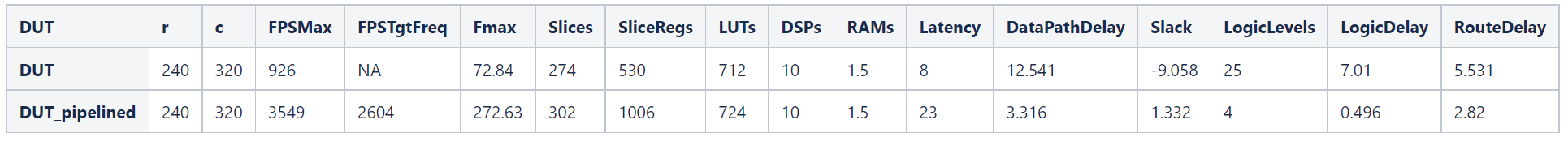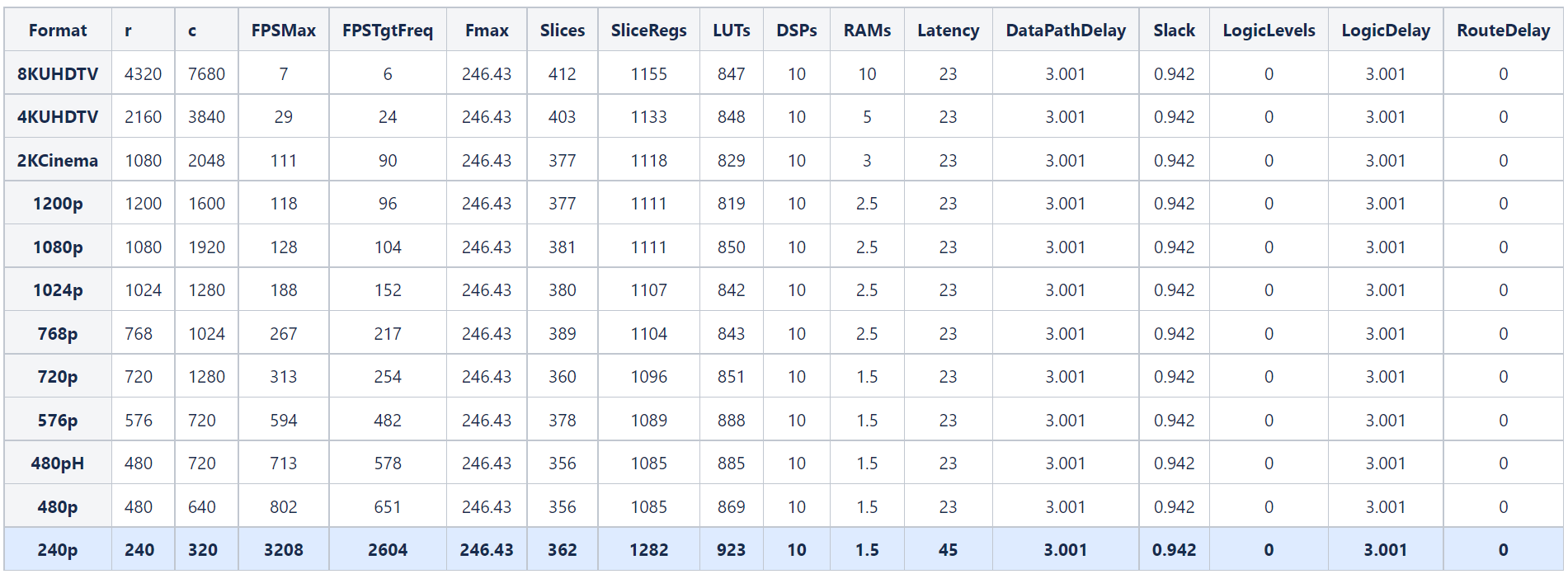# Synthesize Code for Frame-Based Model

This example shows how you can generate HDL code for a Sobel edge detection frame algorithm and calculate the frames per second of your design by synthesizing the generated code with the Simulink® HDL Workflow Advisor.

In this example, you can optimize the speed of the design by either manually pipelining the algorithm or by applying distributed pipelining.

### Sobel Edge Detection Model

Open the model to see a frame-based implementation of the Sobel edge detection algorithm.

```open_system('FrameBasedEdgeDetectionForHDLCodeGenExample') set_param('FrameBasedEdgeDetectionForHDLCodeGenExample','SimulationCommand','Update') ```The model consists of two designs under test (DUT) and a testbench. The `DUT `subsystem contains a MATLAB® Function block that implements a Sobel edge detection algorithm using `hdl.npufun`.

```function [O, E] = edgeDetectionAndOverlay(I) E = hdl.npufun(@sobel_kernel, [3 3], I); O = hdl.npufun(@mix_kernel, [1 1], E, I); end function e = sobel_kernel(in) u = fi(in); hGrad = u(1) + fi(2)*u(2) + u(3) - (u(7) + fi(2)*u(8) + u(9)); vGrad = u(1) + fi(2)*u(4) + u(7) - (u(3) + fi(2)*u(6) + u(9)); hGrad = bitshift(hGrad, -3); % Divide by 8 vGrad = bitshift(vGrad, -3); % Divide by 8 thresholdValueSq = fi(49); % Threshold parameter e = (hGrad*hGrad + vGrad*vGrad) > thresholdValueSq; end function O = mix_kernel(E, I) alpha = fi(0.8); % Parameter for combining images scaleE = E*fi(255,0,8,0); O = scaleE * (fi(1)-alpha) + I*alpha; end ```

The `DUTPipelined `subsystem contains a MATLAB Function block with a manually pipelined version of the previous algorithm that uses the `coder.hdl.pipeline` pragma. For example, you can modify the `mix_kernel` function to insert pipeline registers for the overlay operation.

```function O = mix_kernel(E, I) alpha = fi(0.8); % Parameter for combining images scaleE = E*fi(255,0,8,0); scaleEdelay = coder.hdl.pipeline(scaleE,2); O1 = scaleEdelay*(1-alpha); O1delay = coder.hdl.pipeline(O1,2); O2 = I*alpha; O2delay = coder.hdl.pipeline(O2,4); O = O1delay + O2delay; end ```

### Perform FPGA Synthesis and Analysis

Use the HDL Workflow Advisor to synthesize the DUTs and compare the resources and timing achieved by each of the implementations. To generate HDL code and run synthesis on your design using the HDL Workflow Advisor, see HDL Code Generation and FPGA Synthesis from Simulink Model.

This table shows the results achieved for each DUT subsystem when you use these settings in the HDL Workflow Advisor:

• Synthesis Tool: `Xilinx Vivado`

• Family: `Zynq`

• Device: `xc7z045`

• Package: `ffg900`

• Speed: `-2`

• Target Frequency: `200`

The `FPSMax` and `FPSTgtFreq` columns correspond to the average frames per second (FPS) at the output when using the maximum achievable frequency (`Fma`x) and the Target Frequency (`200 MHz`), respectively, and `r `and `c` correspond to the number of rows and columns of the frame. To calculate the average frames per second, you can use this equation:

$\mathrm{FPS}=\frac{\mathrm{Frequency}\text{\hspace{0.17em}}\left(\mathrm{Hz}\right)}{\mathit{r}\cdot \mathit{c}}$.Converting a frame-based algorithm to a sample-based algorithm requires more time to process each pixel than the frame-based version. This conversion adds additional latency between the input and output frames. In this example, the first pixel of the manually pipelined DUT output frame is available after an initial latency of 321 valid input pixels, plus the latency of 23 clock cycles reported in the table. For more information, see HDL Code Generation from Frame-Based Algorithms.

### Optimized Speed by Using Speed Optimizations

You can use the distributed pipelining and the adaptive pipelining optimizations from HDL Coder™ with the frame-to-sample optimization to reduce the critical path of the design and achieve higher clock speed hardware for the `DUT` subsystem. Distributed pipelining moves existing design delays and pipeline registers in your design to reduce the critical path and adaptive pipelining inserts pipeline registers to blocks in the design that could result in area or speed optimizations. For this example, add input and output pipelines for distributed pipelining to redistribute and enable adaptive pipelining to obtain an optimal result. For more information on distributed pipelining and adaptive pipelining, see Distributed Pipelining and Adaptive Pipelining respectively.

Enable distributed pipelining, adaptive pipelining, and set the MATLAB Function block input and output pipeline registers to 16 by entering:

```hdlset_param('FrameBasedEdgeDetectionForHDLCodeGenExample', 'DistributedPipelining', 'on'); hdlset_param('FrameBasedEdgeDetectionForHDLCodeGenExample', 'AdaptivePipelining', 'on'); hdlset_param('FrameBasedEdgeDetectionForHDLCodeGenExample/DUT/MATLAB Function', 'DistributedPipelining', 'on'); hdlset_param('FrameBasedEdgeDetectionForHDLCodeGenExample/DUT/MATLAB Function', 'OutputPipeline', 16); hdlset_param('FrameBasedEdgeDetectionForHDLCodeGenExample/DUT/MATLAB Function', 'InputPipeline', 16); ```

This table shows the resources and timing achieved when using distributed pipelining for different video formats. The highlighted row corresponds to the video format used in the previous section.For all video formats, the synthesis results show a clock frequency of 246.43 MHz, indicating that the timing constraints are met.

### Optimized Speed by Modifying the Samples per Cycle

You can vary the video size and achieve higher FPS by using the SamplesPerCycle parameter in the Configuration Parameters window:

$\mathrm{FPS}=\frac{\mathrm{Frequency}\text{\hspace{0.17em}}\left(\mathrm{Hz}\right)}{\mathit{r}\cdot \left(\frac{\mathit{c}}{\mathrm{SamplesPerCycle}}\right)}$.

This table shows the FPS obtained for the `DUTPipelined` subsystem (manually pipelined) when you vary the size of the frames and the Samples Per Cycle parameter. For more information on the Samples Per Cycle parameter, see Frame to Sample Conversion Parameters.